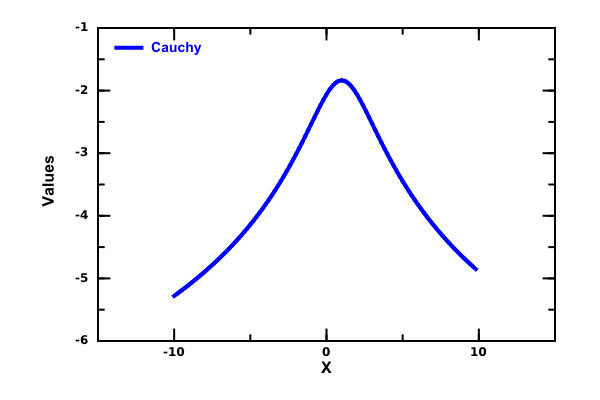# DMelt:Statistics/2 Distribution Functions

Limitted access. First login to DataMelt if you are a full DataMelt member. Then login to HandWiki as a user.

# Distribution functions

Many useful distribution functions can be found incern.jet.stat.Probability package. The package contains numerical integration of certain probability distributions. Below we will show how to use the normal distribution which is very useful distribution in many statistical analyses.

In the example below we will compute the probability that our random outcome is within a specified interval using the normal distribution. The code below returns the area under the normal probability density function, integrated from minus infinity to -1.17 (assumes mean is zero, variance is one).

from cern.jet.stat.Probability import *
print normal(-1.17)

For the two-sided case, one can multiply the result by 2.

Let us consider two other methods: Here an approach based on the javanpst class:

from javanpst.distributions.common.continuous import NormalDistribution
p=NormalDistribution()
print "1 sigma=",p.computeCumulativeProbability(1)
print "2 sigma=",p.computeCumulativeProbability(2)
print "\n Inverse 0.95 sigma. Method 1=",p.inverseNormalDistribution(0.95)

Alternatively, use Apache common math and itsorg.apache.commons.math3.distribution.NormalDistribution class:

from org.apache.commons.math3.distribution import NormalDistribution
p=NormalDistribution()
print "Inverse 0.95 sigma. Method 2=",p.inverseCumulativeProbability(0.95)

# Test distributions

You can find a number of statistical distributionsjavanpst.distributions.tests.package-summary

# Plotting distribution functions

Now let us show how to plot density functions. This is relatively easy if you already read this manual before. Here is a simply code to plot the Cauchy distribution function:

The image of the Cauchy function generated by this code is shown below: